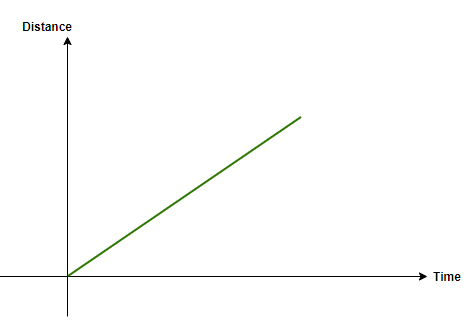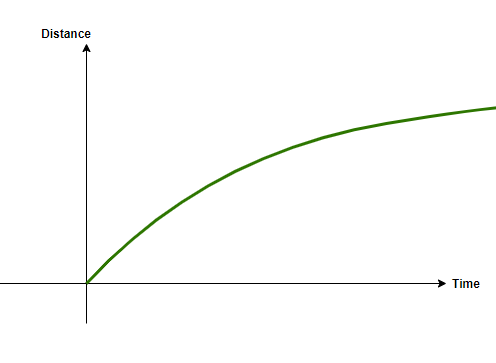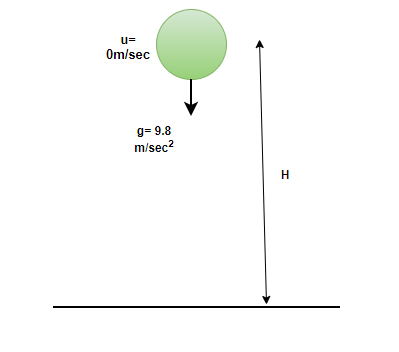GeeksforGeeks App
Open AppBrowser
Continue

## Related Articles

• CBSE Class 11 Physics Notes

# Solving Problems Based on Free Fall

An object when in rest position has energy stored in it and when the object starts to move, it is said that the object is in motion. An object in motion has Kinetic energy, and it is not generated, it is obtained by simply converting the energy object had when it was in rest position. Motion can be defined in different ways, 1-D motion or one-dimensional motion is when the object moves in 1 co-ordinate only, for example, A boy riding his cycle in a straight line. 2-D or two-dimensional motion is when the object moves in 2 co-ordinates, mostly in both x and y co-ordinates, for example, children playing and running around in different directions. 3-D or three-dimensional motion is observed when an object moves in all three dimensions, for example, an airplane or a fighter jet moves in all three directions.

There are some special types of motion like Circular Motion, Parabolic motion, Free fall. However, these also come under the category of the ones mentioned above, that is, Circular motion is a 2- D motion, Parabolic motion is 2-D as well, Free fall is One dimensional. The most basic type of motion to learn and understand is 1-D motion. Let’s learn about 1-D motion in more detail,

### Motion in a Straight Line

A body travelling in a straight line and in one direction has motion in a straight line. Examples can be a car moving in a straight line or an object free falling. The body is known to have uniform motion in a straight line if it travels equal distance in per unit time. For example, if a car travels 3 meters every second, it is covering equal distance every second, the speed of the body is 3m/sec.Uniform motion in a straight line

The other case can be having uniform acceleration, in this case, the body has constant acceleration, the speed changes uniformly.

The body/object is known to have non-uniform motion in a straight line if it travels unequal distances in equal intervals of time. For example, A car traveling 2 meters for the first second and 3 meters for the next second.Non- uniform motion in a straight line

### Free Fall

Free fall of an object is defined when the object is only under the influence of the force of gravity and no other force is acting upon it. In real life, an object when undergoes free fall, it also experiences other forces like air friction, etc. Hence, it will not be considered a Free Fall. Instances of Free fall can be the moon revolving around the sun, as it only experiences force under• First Equation of motion

Since, during Free fall, the initial velocity of the object is 0m/sec and the acceleration acted upon is the acceleration due to gravity (g= 9.8 m/sec2). the equation will look like,

v= u+ at

u=0m/sec, a=g= 9.8m/sec2

v = gt

• Second Equation of motion

As mentioned above, the second equation of motion under free fall shall be,

S = ut+ 1/2(at2)

S = H, u=0m/sec, a= g= 9.8 m/sec2

H = 1/2(gt2)

• Third Equation of motion

The third equation of motion under free fall,

v2 = u2+ 2aS

S= H, u=0m/sec, a= g= 9.8m/sec2

v2 = 2gH

Table to show the Final Velocities, distance covered, in every second of a Free Fall,

### Sample Problems

Question 1: What happens when an object undergoes free fall?

When an object undergoes free fall, it starts with zero initial velocity, and keeps on increasing its velocity with a rate of 9.8 m/sec, hence, it experiences an acceleration of 9.8 m/sec2 which is also known as acceleration due to gravity.

Question 2: What is the final velocity of the ball if it is dropped from a certain height and takes 10 seconds to reach the ground. The air resistance is not taken into account. [take g= 10 m/sec2]

Solution:

Applying first equation of motion under free fall,

u= 0m/sec, S=H, a= g= 10m/sec2 (given)

v= u+ at

v= gt

v= 10× 10

v= 100m/sec

Question 3: Rohan dropped his ball from a height of 5 meters and then he ran downstairs really fast to see if he can catch the ball, he takes 1 minute to reach the ground. Will he be able to catch the ball, please note that the air friction is neglected.

Solution:

Applying second equation of motion under free fall,

S= ut+ 1/2(at2)

u=0 m/sec, a= g= 9.8m/sec2, S=H

H= 1/2(gt2)

5= 1/2(9.8× t2)

t2= 10/9.8

t= 1.01 second

As it is very clear that Rohan takes 1 whole minute to reach the ground while the ball reaches the ground in 1.01 second. Hence, there is no way he will be able to catch the ball.

Question 4: If an object undergoes Free fall, what will be the velocity of the object on the 10th second?

Solution:

Applying first equation of motion under free fall,

v= gt

v= 9.8× 10

v= 98m/sec

Therefore, on the 10th second, the velocity of the object will be 98m/sec.

Question 5: Find the height from which a toy is dropped, if it goes in free fall state and the final velocity achieved by the toy is 20m/sec.

Solution:

Applying third equation of motion,

v2= u2+ 2aS

Under free fall,

u= 0m/sec, a= g= 9.8m/sec2, S=H

v2= 2gH

202= 2× 9.8× H

400 = 19.6× H

H= 20.40 meters.

My Personal Notes arrow_drop_up
Related Tutorials